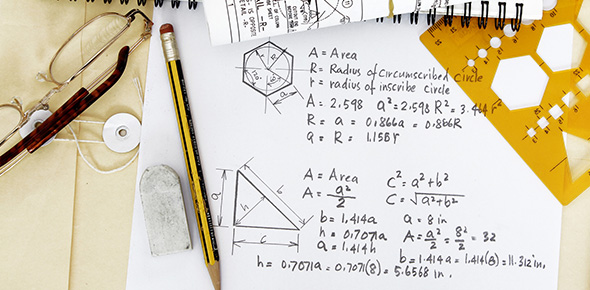# Section 8.4 - Surface Area Of Right Pyramids And Cones

5 Questions | Total Attempts: 320SettingsComplete the following questions.

• 1.
What is the surface area of a square pyramid with a height of 15cm and a base side length of 16cm?
• A.

576 cm^2

• B.

800 cm^2

• C.

960 cm^2

• D.

1344 cm^2

• 2.
A trophy in the shape of a pyramid has a regular pentagonal base with a perimeter that measures 95 cm.  The pyramid has a slant height of 32 cm, and the distance from the centre of the base to the midpoint of each side is 13 cm.  What is the surface area of the trophy?
• A.

1615 cm^2

• B.

1881 cm^2

• C.

2138 cm^2

• D.

2698 cm^2

• 3.
What is the surface area of a cone with height 8.0m and radius 3.0m?
• A.

52.3 m^2

• B.

98.9 m^2

• C.

108.3 m^2

• D.

268.5 m^2

• 4.
Ana wants to wrap a conical candle that has a radius of 5,9 cm, a height of 7.3 cm, and a slant height of 9.4 cm.  How much wrapping paper will she need?
• A.

164.8 cm^2

• B.

202.4 cm^2

• C.

211.2 cm^2

• D.

283.4 cm^2

• 5.
What is the surface area of a square pyramid with a height of 39.4 mm and a base area of 676.0 mm^2
• A.

2834.0 mm^2

• B.

3130.0 mm^2

• C.

4316.0 mm^2

• D.

4992.0 mm^2

Related TopicsBack to top